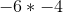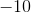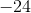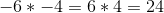## Example Questions

### Example Question #4 : Negative Numbers

If a = –2 and b = –3, then evaluate a3 + b2

5

1

9

8

17

1

Explanation:

When multiplying negative numbers, we get a negative answer if there are an odd number of negative numbers being multiplied.  We get a positive answer if there are an even number of negative numbers being multiplied.

a3 + b2 becomes (–2)3 + (–3)2 which equals –8 + 9 = 1

### Example Question #1 : How To Multiply Negative Numbers

Evaluate:

–3 * –7

4

21

–21

10

–10

21

Explanation:

Multiplying a negative number and another negative number makes the product positive.

### Example Question #6 : Negative Numbers

Evaluate.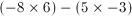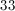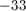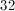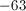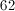Explanation:Multiplying a negative and a positive number creates a negative product: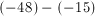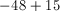### Example Question #7 : Negative Numbers

Solve.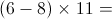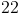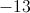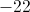Explanation: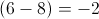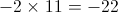A negative number multiplied by a positive number will always be negative.

### Example Question #1 : Negative Numbers

Evaluate 3x3 + x2 if x = 2

28

22

14

20

20

Explanation:

When multiplying a negative number an odd number of times, the answer is negative.  When multiplying a negative number an even number of times, the answer is positive.  Order of operations also applies:  Parentheses, Exponents, Multiplication and Division, Addition and Subtraction, from left to right.  A mnemonic to remember the order of operations is “Please excuse my dear Aunt Sally.”

3(2)3 + (2)2

= 3(8) + (4)

= 24 + 4

= 20

### Example Question #9 : Negative Numbers

Simplify the following expression: (–4)(2)(–1)(–3)

–16

24

–24

12

–24

Explanation:

First, we multiply –4 and 2. A negative and a positive number multiplied together give us a negative number, so (–4)(2) = –8. A negative times a negative is a positive so (–8)(–1) = 8. (8)(–3) = –24.

### Example Question #10 : Negative Numbers

Evaluate the following: